• Slides: 154. 1 Graphing Quadratic Functions Standard FormQuadratic Function �Highest degree is 2. �Its graph is called a parabola. quadratic term linear term constant term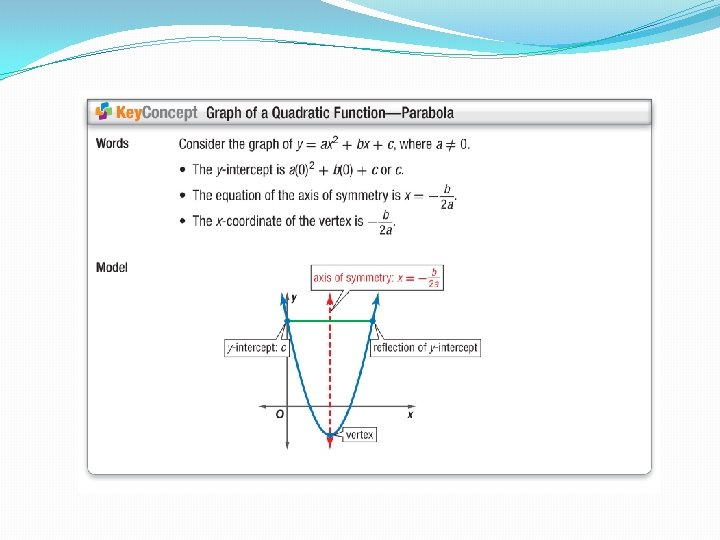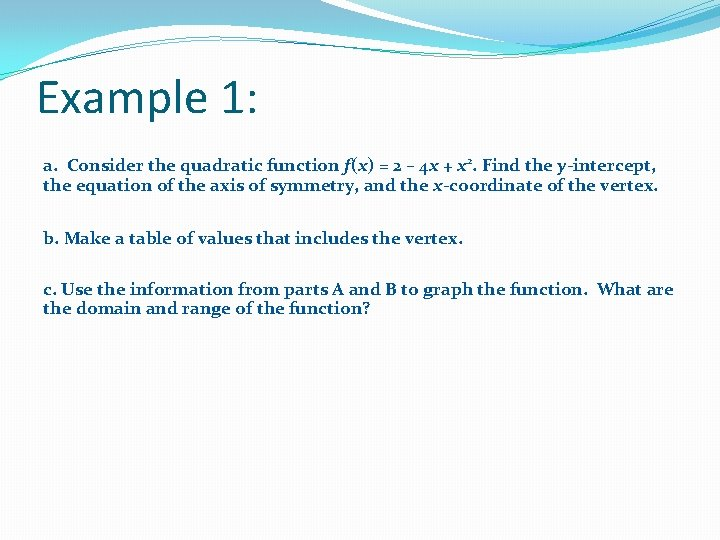Example 1: a. Consider the quadratic function f(x) = 2 – 4 x + x 2. Find the y-intercept, the equation of the axis of symmetry, and the x-coordinate of the vertex. b. Make a table of values that includes the vertex. c. Use the information from parts A and B to graph the function. What are the domain and range of the function?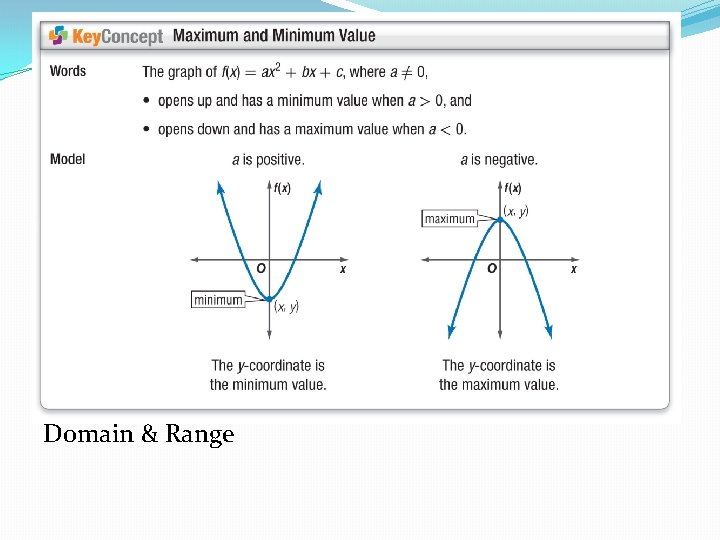Domain & Range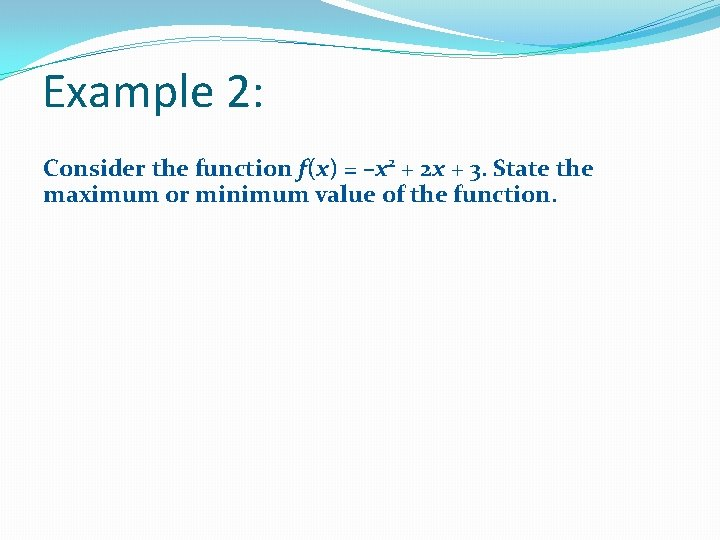Example 2: Consider the function f(x) = –x 2 + 2 x + 3. State the maximum or minimum value of the function.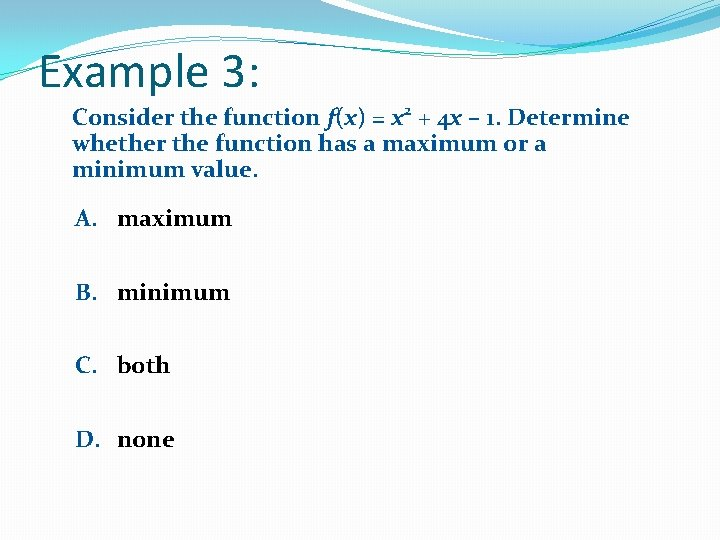Example 3: Consider the function f(x) = x 2 + 4 x – 1. Determine whether the function has a maximum or a minimum value. A. maximum B. minimum C. both D. none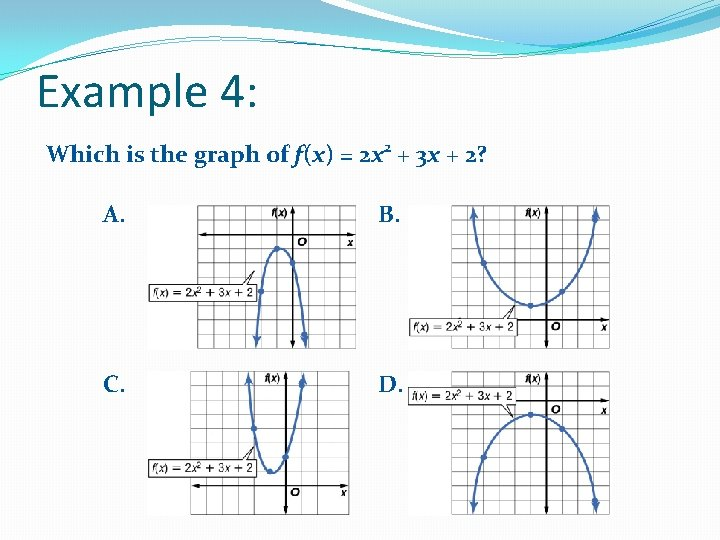Example 4: Which is the graph of f(x) = 2 x 2 + 3 x + 2? A. B. C. D.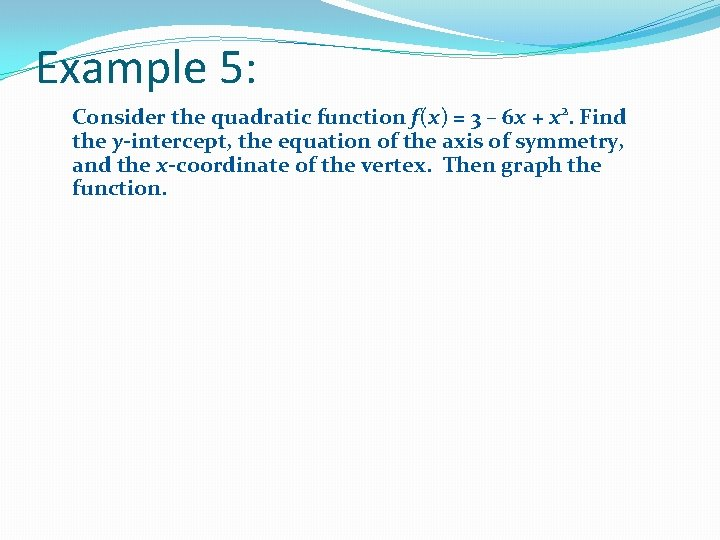Example 5: Consider the quadratic function f(x) = 3 – 6 x + x 2. Find the y-intercept, the equation of the axis of symmetry, and the x-coordinate of the vertex. Then graph the function.Example 6: Consider the function f(x) = x 2 + 4 x – 1. What are the domain and range of the function?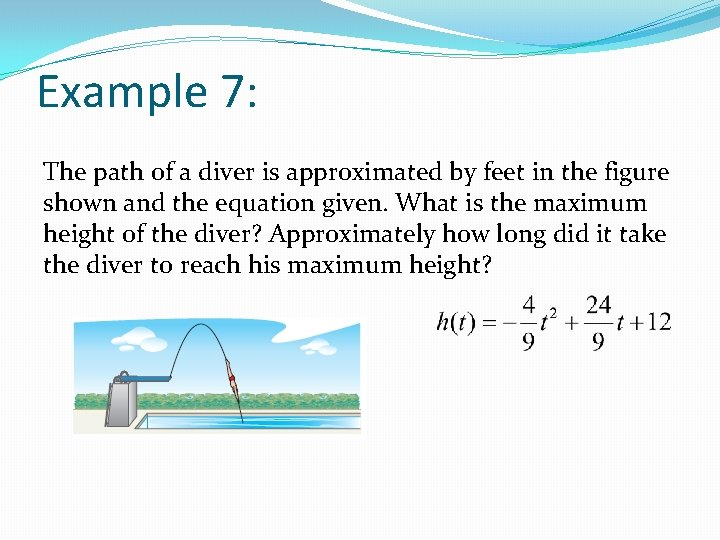Example 7: The path of a diver is approximated by feet in the figure shown and the equation given. What is the maximum height of the diver? Approximately how long did it take the diver to reach his maximum height?Example 8: a. ECONOMICS A souvenir shop sells about 200 coffee mugs each month for \$6 each. The shop owner estimates that for each \$0. 50 increase in the price, he will sell about 10 fewer coffee mugs per month. How much should the owner charge for each mug in order to maximize the monthly income from their sales?Example 8: b. What is the maximum monthly income the owner can expect to make from these items?Calculator Steps Finding the Vertex - Input equation into y= - 2 nd >> trace >> minimum or maximum - Min or Max will depend on the direction of the parabola - Move the flashing cursor to the left of the vertex and press enter - Repeat for the right side of the vertex - Press enter for a 3 rd time to have calculator “Guess? ” the vertex point.Calculator Steps Finding the X-intercept - Input equation into y= - 2 nd >> trace >> zero - Move the flashing cursor to the left of the x-intercept and press enter - Repeat for the right side of the x-intercept - Press enter for a 3 rd time to have calculator “Guess? ” the vertex point.